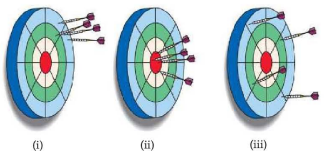# Problem: The following dartboards illustrate the types of errors often seen when one measurement is repeated several times. The bull's-eye represents the "true value," and the darts represent the experimental measurements. Which board best represents each of the following scenarios: (a) measurements both accurate and precise? [Section 1.5]

###### FREE Expert Solution

We’re being asked to identify the board that represents measurements as both accurate and precise.

Recall that:

• Accuracy refers to how close the measurement is to the ‘true/actual value’.

• Precision refers to how close the measurements are to each other.

For this problem, the true value is the bull’s-eye of the dartboard

Let’s analyze each board:###### Problem Details

The following dartboards illustrate the types of errors often seen when one measurement is repeated several times. The bull's-eye represents the "true value," and the darts represent the experimental measurements. Which board best represents each of the following scenarios: (a) measurements both accurate and precise? [Section 1.5]